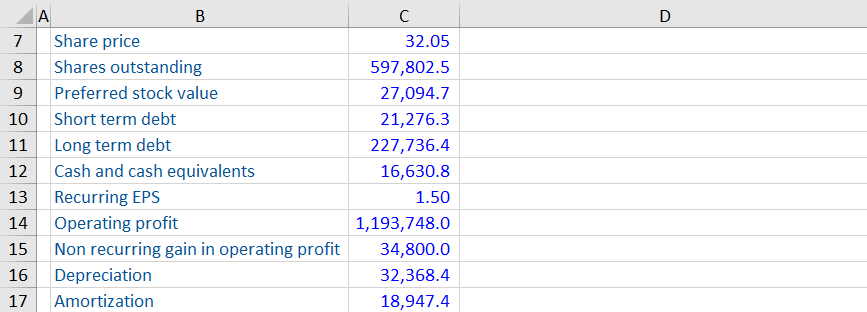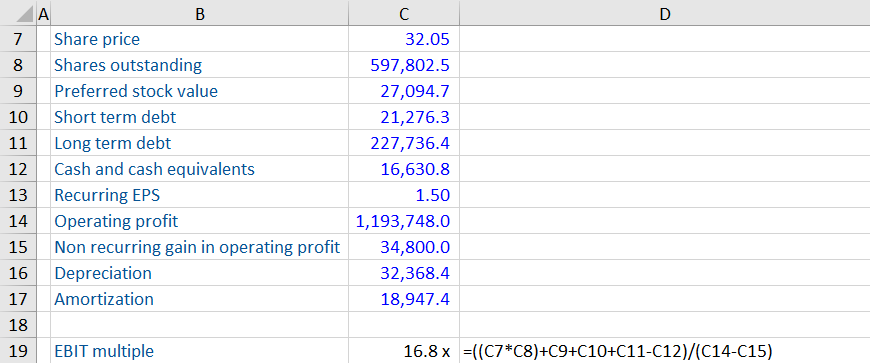## What is EV/EBIT?

Enterprise Value to EBIT (EV/EBIT), also called EV Multiple is a ratio used to to value a company and provide useful comparisons between similar companies. It is used in trading comparables analysis and uses the EBIT of a company as the driver of its value.

As with all valuation multiples, the value must be consistent with the value driver. EBIT is unaffected by a company’s capital structure and therefore is a driver of EV.

The multiple should only be used to compare similar businesses as different industries each have their own benchmark.

## Key Learning Points

• EV / EBIT is a commonly used valuation multiple to calculate the value of a company
• EBIT is a calculated number and represents the recurring operating profit
• EBIT is unaffected by capital structure and is therefore a driver of enterprise value (EV)
• It is a common multiple and factors in the operating efficiency of a company (i.e. its ability to turn sales into operating profit)
• Enterprise value is the value of the core business before any financial assets and the deduction of debt

## The Formula

The multiple is calculated as:

EV / EBIT = Enterprise value / EBIT

Enterprise Value (EV) is the value number. This the value of the core business before any financial assets and the deduction of debt. In other words, it is unaffected by a company’s capital structure. In its simplest form, EV is calculated as:

EV = Equity value + Debt – Cash & Cash Equivalents

EBIT is a calculated number that represents the recurring operating profit. The operating profit is reported in the income statement and is then cleaned for any non-recurring items. It is unaffected by a company’s capital structure and is therefore a driver of EV.

## Calculating EV / EBIT

Using the following information about Hook Inc, calculate the EBIT, EBITDA and PE multiples.The calculation can be broken down into two parts.

### Calculating Enterprise Value

We can use the the equity to EV bridge to calculate it.

Equity value is calculated as the share price (32.05) multiplied by the number of shares outstanding (597,802.5). Then the other sources of finance must be added, these are: preferred stock value (27,094.7), short term debt (21,276.3) and long term debt (227,736.4). Finally, cash and cash equivalents (16,630.8) is subtracted to equal the EV.

### Calculating EBIT

EBIT is calculated as the recurring operating profit. Operating profit is provided (1,193,748.0) but there is also a non recurring gain in operating profit of 34,800.0. This is already included in the profit number so must therefore be subtracted to arrive at EBIT. The final calculation is summarized below:EBIT multiple is 16.8 x.

Download our Excel workout to see the calculations for the PE multiple and EBITDA multiple.

## Analyzing EBIT Multiple

The EBIT multiple is a commonly used valuation multiple and uses operating profit as a driver of a company’s value. Earnings drive value and EBIT focuses on the core earnings generated by a business so is a great comparison tool.

Many analyst also use the EBTIDA multiple which is before the impact of depreciation and amortization. This is useful when comparing capital intensive companies with varying depreciation policies. EBIT does include these as expenses and recognizes them as real expenses as they represent the utilization of company assets. For less capital intensive companies, EBIT multiple provides a good comparison and will be close to EBITDA.

Higher growth rates impact the multiple and increases the EV multiple, how? The value number or the EV is the present value of all the future cash flows so includes 100% of the company’s growth. However, the earnings, or in this case the EBIT will either be a historical number or one or two years forward and so it won’t reflect the long-term future growth profile. So if investors think a stock will grow strongly then they will price it into the value number, but the earnings will take time to catch up. This is one reason why stocks like Tesla trade on such high multiples.